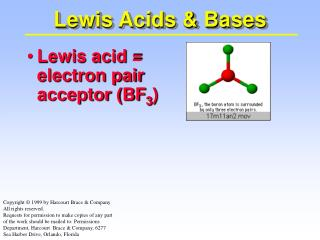# Lewis Acids & Bases - PowerPoint PPT PresentationDownload PresentationLewis Acids & Bases

Lewis Acids & Bases
Download Presentation## Lewis Acids & Bases

- - - - - - - - - - - - - - - - - - - - - - - - - - - E N D - - - - - - - - - - - - - - - - - - - - - - - - - - -
##### Presentation Transcript

1. Lewis Acids & Bases • Lewis acid = electron pair acceptor (BF3) Copyright © 1999 by Harcourt Brace & Company All rights reserved. Requests for permission to make copies of any part of the work should be mailed to: Permissions Department, Harcourt Brace & Company, 6277 Sea Harbor Drive, Orlando, Florida

2. Lewis Acids & Bases • Lewis acid = electron pair acceptor (BF3) • Lewis base = electron pair donor (NH3)

3. Lewis Acids & Bases A Lewis acid and base can interact by sharing an electron pair.

4. + Lewis Acids & Bases A Lewis acid and base can interact by sharing an electron pair. Formation of hydronium ion is an excellent example.

5. Lewis Acids & Bases Other good examples involve metal ions.

6. Lewis Acids & Bases Other good examples involve metal ions. Such bonds as the H2O ---> Co bond are often called COORDINATE COVALENT BONDS because both electrons are supplied by one of the atoms of the bond.

7. Lewis Acids & Bases The combination of metal ions (Lewis acids) with Lewis bases such as H2O and NH3 ------> COMPLEX IONS All metal ions form complex ions with water —and are of the type [M(H2O)x]n+ where x = 4 and 6. [Cu(NH3)4]2+

8. Lewis Acids & Bases Add NH3 to light blue [Cu(H2O)4]2+ ------> light blue Cu(OH)2 and then deep blue [Cu(NH3)4]2+

9. Lewis Acids & Bases [Ni(H2O)6]2+ + 6 NH3 ---> [Ni(NH3)6]2+ + DMG

10. Lewis Acids & Bases The Fe2+ in heme can interact with O2 or CO in a Lewis acid-base reaction.

11. Lewis Acids & Bases Many complex ions containing water undergo HYDROLYSIS to give acidic solutions.

12. Lewis Acids & Bases Many complex ions containing water undergo HYDROLYSIS to give acidic solutions. [Cu(H2O)4]2+ + H2O ---> [Cu(H2O)3(OH)]+ + H3O+

13. Lewis Acids & Bases Many complex ions containing water undergo HYDROLYSIS to give acidic solutions. This explains why water solutions of Fe3+, Al3+, Cu2+, Pb2+, etc. are acidic.

14. Lewis Acids & Bases This explains AMPHOTERICnature of some metal hydroxides. Al(OH)3(s) + 3 H+ --> Al3+ + 3 H2O Here Al(OH)3 is a Brønsted base. Al(OH)3(s) + OH- --> Al(OH)4- Here Al(OH)3 is a Lewis acid.

15. Lewis Acids & Bases This explains AMPHOTERICnature of some metal hydroxides. Al(OH)3(s) + 3 H+ --> Al3+ + 3 H2O Here Al(OH)3 is a Brønsted base. Al(OH)3(s) + OH- --> Al(OH)4- Here Al(OH)3 is a Lewis acid.

16. Amphoterism of Al(OH)3 Al(OH)3 on right Add NaOH Add HCl See Kotz/Treichel, page 830

17. -0.75 +1.5 -0.75 Neutral Lewis Acid Carbon dioxide is a neutral Lewis acid.

18. Lewis Acids & Bases Many complex ions are very stable. Cu2+ + 4 NH3[Cu(NH3)4]2+ K for the reaction is called Kformation or a “formation constant” Here K = 6.8 x 1012. Reaction is strongly product-favored.

19. Lewis Acids & Bases Formation of complex ions explains why you can dissolve a ppt. by forming a complex ion. AgCl(s) + 2 NH3 Ag(NH3)2+ + Cl- AgCl(s)

20. Lewis Acids & Bases Formation of complex ions explains why you can dissolve a ppt. by forming a complex ion. AgCl(s) Ag+ + Cl- Ksp = 1.8 x 10-10 Ag+ + 2 NH3 --> Ag(NH3)2+ Kform = 1.6 x 107 ------------------------------------- AgCl(s) + 2 NH3Ag(NH3)2+ + Cl- Knet = Ksp • Kform = 2.9 x 10-3